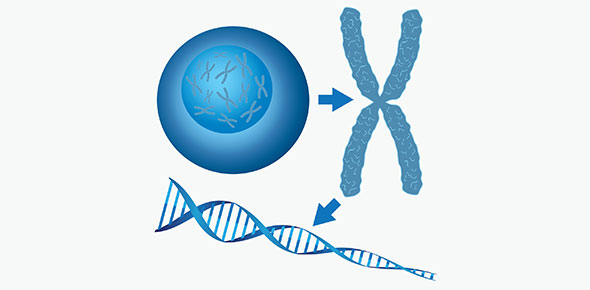# Genetics Test Haldorson

28 Questions | Total Attempts: 87SettingsThere are a number of reasons why studying our genetics would be beneficial to us, but perhaps the most important one involves the ability to understand our genetic variation and heredity, to see if we are likely to suffer from diseases like cancer and heart disease as a result of our parents or grandparents have suffered from the same thing. Think you know enough about genetics? Find out now!

Related Topics
• 1.
The passing on of traits from an organism to its offspring is known as
• A.

Biology

• B.

Chemistry

• C.

Heredity

• D.

Zoology

• 2.
The basic units that pass on traits are called:
• A.

Genes

• B.

Cells

• C.

Mitochondria

• D.

Genotypes

• 3.
The visible characteristics of an organism are called:
• A.

Cells

• B.

Genotypes

• C.

Phenotypes

• D.

Chromosomes

• 4.
Hh is an example of an organism's
• A.

Cell

• B.

Genotype

• C.

Phenotype

• D.

Chromosome

• 5.
When one gene in a pair totally dominates another gene, the genes are said to show:
• A.

Heterozygous

• B.

Complete dominance

• C.

Incomplete dominance

• D.

Meiosis

• 6.
A trait that is the "weaker" of 2 traits is called
• A.

Incomplete

• B.

Dominant

• C.

Recessive

• D.

Heterozygous

• 7.
Sex cells(egg and sperm) are produced by the process of:
• A.

Meiosis

• B.

Mitosis

• C.

Fertilization

• D.

Complete dominance

• 8.
If human cells have 46 chromosomes, the sex cells must have_________ chromosomes.
• A.

46

• B.

92

• C.

64

• D.

23

• E.

42

• 9.
If "T" represents the gene for spiked tail and "t" represents the gene for no spikes, which of the following would represent a heterozygous individual?
• A.

TT

• B.

Tt

• C.

Tt

• D.

T

• E.

T

• 10.
If 2 dragons that are "Tt" for tail genes are crossed and have 100 baby dragons, how many of them will have the genotype "Tt" ?
• A.

25

• B.

50

• C.

2

• D.

4

• E.

0

• 11.
If 2 dragons that are "Tt" for tail genes are crossed and had 4 offspring, how many would have no spikes?  ( TT = spikes   Tt = spikes   tt = no spikes)
• A.

1

• B.

2

• C.

3

• D.

4

• 12.
The rod shaped structures located in the nucleus of every cell of an organism are called:
• A.

Chromosomes

• B.

Cell membranes

• C.

Genes

• D.

Chloroplasts

• E.

Fishing poles

• 13.
If red flower color "R" is incompletely dominant over white flower color "r" then what would be the color of an individual that has a genotype of "RR"?
• A.

Red

• B.

White

• C.

Pink

• D.

Black

• 14.
R =redr = whiteincompletely dominantwhat would the color be of an individual that has a genotype of "rr"?
• A.

Red

• B.

White

• C.

Pink

• D.

Black

• 15.
R =redr = whiteincompletely dominantwhat would the color be of an individual that has a genotype of "Rr"?
• A.

Red

• B.

White

• C.

Pink

• D.

Black

• 16.
What would be the expected phenotypic ratio of a cross between "Rr" and "Rr"?R =redr = whiteincompletely dominant
• A.

1 red : 3 white

• B.

2 white : 2 red

• C.

1red : 2 white : 1 black

• D.

1 red : 2 pink : 1 white

• 17.
In humans, which chromosome combination is that of a male?
• A.

XX

• B.

XY

• C.

YY

• D.

YMCA

• 18.
Which one of the female DRAGON'S chromosomes had no genes?
• A.

X

• B.

Y

• C.

Z

• D.

1a

• E.

• 19.
Which of the following would be a genotype of a homozygous recessive individual?
• A.

AaBB

• B.

AABB

• C.

AaBb

• D.

AABb

• E.

Aabb

• 20.
The chart used by scientists to show possible combinations resulting from a cross between two organisms is a:
• A.

Punnett square

• B.

Pedigree

• C.

Phenotypic diagram

• D.

Genotypic diagram

• E.

Pie chart

• 21.
Alleles are:
• A.

Different forms of chromosomes

• B.

Different forms of DNA

• C.

Different forms of genes

• D.

Different forms of cells

• E.

Always homozygous

• 22.
How many of the offspring have horns?
• A.

1

• B.

2

• C.

3

• D.

4

• E.

None of the offspring have horns

• 23.
What is the most likely genotype of the mother dragon?
• A.

HH

• B.

Hh

• C.

Hh

• D.

Horns

• E.

No horns

• 24.
What is the genotype of the father?
• A.

HH

• B.

Hh

• C.

Hh

• D.

Horns

• E.

No horns

• 25.
Letter B represents a:
• A.

Chromosome

• B.

Gene

• C.

Trait

• 26.
Letter "A" represents a:
• A.

Chromosome

• B.

Gene

• C.

Trait

• 27.
The letter "A" represents a:
• A.

Cell

• B.

Nucleus

• C.

Gene

• D.

Chromosome

• 28.
Letter "B" is pointing to a:
• A.

Cell

• B.

Nucleus

• C.

Chloroplast

• D.

Chromosome